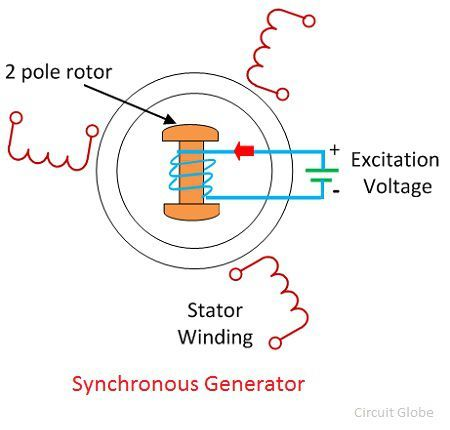# Synchronous Generators

Definition: The synchronous generator or alternator is an electrical machine that converts the mechanical power from a prime mover into an AC electrical power at a particular voltage and frequency. The synchronous motor always runs at a constant speed called synchronous speed.

## Working Principle of Synchronous Generator

The synchronous generator works on the principle of Faraday laws of electromagnetic induction. Electromagnetic induction states that electromotive force induced in the armature coil if it is rotating in the uniform magnetic field. The EMF will also be generated if the field rotates and the conductor becomes stationary. Thus, the relative motion between the conductor and the field induces the EMF in the conductor. The wave shape of the induced voltage is always a sinusoidal curve.

### Construction of Synchronous Generator

The rotor and stator are the rotating and the stationary part of the synchronous generator. They are the power generating components of the synchronous generator. The rotor has the field pole, and the stator consists of the armature conductor. The relative motion between the rotor and the stator induces the voltage between the conductor.### Applications of Synchronous Generator

The three-phase synchronous generators have many advantages in generation, transmission, and distribution. The large synchronous generators use in the nuclear, thermal, and hydropower system for generating the voltages.

The synchronous generator with 100MVA power rating uses in the generating station. The 500MVA power rating transformer use in the super thermal power stations. The synchronous generators are the primary source of electrical power. For the heavy power generation, the stator of the synchronous generator design for voltage ratings between 6.6 kV to 33 kV.

### 3 thoughts on “Synchronous Generators”

1. how the synchronous generator runs at the constant speed means synchronous speed. please tell me